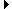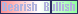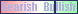Advertising space available Members AreaSign Up | Log In
 Free Level 2 QuotesStock AnalysisCorrelation Trading PlatformCorrelationsMembership Benefits

SMA
 Lookup a different indicator.

 Indicator Usage Description Example sma sma[x,n] OPERATOR (INDICATOR or POSITIVE NUMBER) , where x is a positive integer >= 2 and n is optional and represents the number of days ago the result is computed (n=0 is the default value and represents the current end of day, n=1 is the previous end of day, etc.) Returns the x-day simple moving average closing price from n days ago. (sma > sma)

Introduction:

The 'sma' indicator is used in indicator formula construction to narrow the stock pre-screener results to include only those stocks that have a simple moving average stock price restricted to the parameters set for the sma indicator.

Calculation:

Add up each of the last x-day closing stock prices then divide the sum by x.

Example:

Below is a chart of Amazon (AMZN) highlighting days where the last daily close was above the 50-day simple moving average AND the previous daily close was below the the 50-day simple moving average.

 Chart of Amazon (AMZN) highlighting days where the last daily close was above the 50-day simple moving average AND the previous daily close was below the the 50-day simple moving average.

INDICATORS IN OUR DATABASE THAT INCLUDE THE SMA INDICATOR...

Click here to view all indicators that include the indicator 'sma' (sorted by AISCORE)

 (vol > 1.5 * avevol) and (close > sma)AISCORE: 1492Indicator ID: 051102155300(smamdir[25,p]) and (close > sma)AISCORE: 1163Indicator ID: 051107215357(smamdir[14,p]) and (close > sma)AISCORE: 1073Indicator ID: 051107215409(vol > 2 * vol) and (close >= sma)AISCORE: 1260Indicator ID: 051125125422(vol > 2 * vol) and (close < sma)AISCORE: 1676Indicator ID: 051125125603 More ->